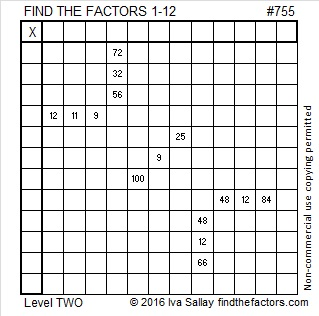# 755 and Level 2

• 755 is a composite number.
• Prime factorization: 755 = 5 x 151
• The exponents in the prime factorization are 1 and 1. Adding one to each and multiplying we get (1 + 1)(1 + 1) = 2 x 2 = 4. Therefore 755 has exactly 4 factors.
• Factors of 755: 1, 5, 151, 755
• Factor pairs: 755 = 1 x 755 or 5 x 151
• 755 has no square factors that allow its square root to be simplified. √755 ≈ 27.4772633.Here’s today’s puzzle:Print the puzzles or type the solution on this excel file: 12 Factors 2016-01-25

—————————————

Here’s a little more about 755:

755 is the sum of consecutive numbers three different ways:

• 377 + 378 = 755; that’s 2 consecutive numbers.
• 149 + 150 + 151 + 152 + 153 = 755; that’s 5 consecutive numbers.
• 71 + 72 + 73 + 74 + 75 + 76 + 77 + 78 + 79 + 80 = 755; that’s 10 consecutive numbers.

Because 5 is one of its factors, 755 is the hypotenuse of Pythagorean triple 453-604-755.

755 is the sum of three squares six different ways:

• 27² + 5² + 1² = 755
• 25² + 11² + 3² = 755
• 25² + 9² + 7² = 755
• 23² + 15² + 1² = 755
• 21² + 17² + 5² = 755
• 19² + 15² + 13² = 755

755 is palindrome 131 in BASE 26; note that 1(26²) + 3(26) + 1(1) = 755.

—————————————This site uses Akismet to reduce spam. Learn how your comment data is processed.